## July 7, 2014

### Thinking of Set in esProc

1.Sequence and Set in esProc

Unlike traditional programming languages, esProc employs set commonly. In fact, the sequence in esProc belongs to the field of set. So it’s quite important to deeply understand the concept of sets when using esProc. Like an integer and a string, the set is a basic data type; it could be a variable value, a computation of an expression, and a return value of a function.

As a data type, esProc provides operators of two sets A and B, like intersection, union, union of set, subtract and so on: A^B，A|B，A&B，A\B.

It is recommended for users to deeply understand and master these set operations. The thinking of sets and good use of data may bring about an easier solution.

The following is an illustration of using set operations to simplify code:

Unlike a set in the sense of mathematics,that in esProc is arranged orderly; there may be duplicates of a member in a set, like that in a sequence or sequence table.In the scope of mathematics, the set Intersection and Union operations are both commutative; in other words, A∩B=B∩A and A∪B=B∪A. However, this commutative property is not valid for esProc because the member order in a sequence for esProc cannot be changed at will. For esProc, the result of the intersection / union operation is required to be arranged according to the order of the left operator.

Because of this ordering feature of sequence members, we should adopt function A.eq(B)to judge whether two sequences have the same members instead of simply using the comparison operator == :

2.Parameters in a Loop Function

With the data type of set, you can handle several operations on the members of set in a single clause, without the need of loop code.Sometimes, the loop functions will not process the set members but the values computed based on the members. In this case, you are allowed to use parameters to represent the formula in a function, in which the "~" represents the current member.For the nested loop functions, "~" is interpreted as a member in an inner sequence; in such case, if you want to reference a member in an outer sequence, name of the outer sequence must headed the "~".The above rule is also applicable to the field reference when ~ is omitted, of which the fields will be
interpreted as fields in an inner RSeq first, if such fields cannot be found in the inner RSeq, esProc will search for them in the outer RSeq.

3.Order of Loop

The arguments in a loop function will be computed according to their order in the original sequence. This is very important.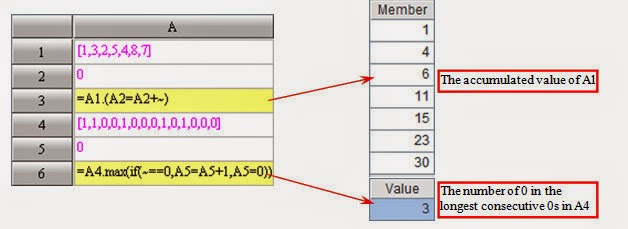In many cases, it is available for a single expression to implement the function which can be achieved by simple loop code.

4.Computation Sequence

Different from such loop functions as sum and avg returning a single aggregate value, a computation sequence function A.(x)returns a set. Often, a new set can also be created by employing computation sequence, in addition to using the basic set operations such as union, intersection and subtract.The execution of an aggregate function with arguments will be divided into two steps:
1) Use arguments to produce computed column
2) Aggregate the result

In other words, A.f(x) = A.(x).f().

The new function is used to return a sequence table by computation sequence.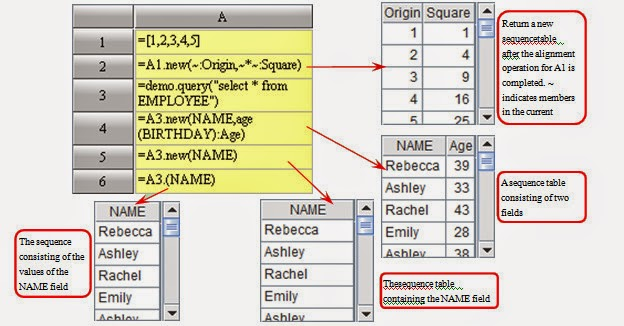In addition, there is a run function for relevant sequence computation. This function returns the original sequences themselves instead of the result of the relevant sequence computation. Generally, this function is used to assign values to fields in a record sequence (sequencetable).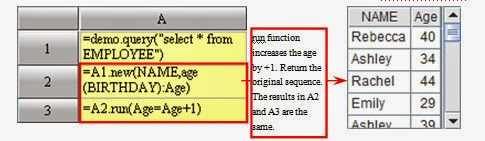5.Impure Sets
esProc has no restriction on the consistency of sequence member types, that is, a sequence may consist of numbers, character strings, and records.

However, in many cases, it would be of little practical significance to arrange data in variable types in one sequence. Therefore, users should not be too concerned about it.

On the other hand, a record sequence – A sequence consisting of records – can consist of records from different sequencetables; this is very convenient.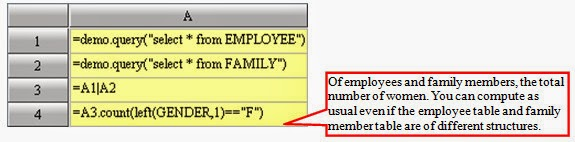Under the environment of esProc, it is not necessary that records in a record sequence originate from the same sequencetable. As long as they have the same field names, the records can be processed uniformly. Here, merits of esProc include simpler program writing, higher efficiency and reduced occupation of memory. For SQL, however, two tables with different structures must be united into a new one by using the UNION clause before making any operations.

6.Sets consisting of Subsets

Since the member of a set in esProc is less restrictive by nature, a set may be a member of another set.If A is a set consisting of other sets, functions A.conj(), A.union(), A.diff(), A.isect() could be employed to compute concatenate, union, difference and intersection between subsets of A.Also, a record sequence may be a member of a sequence.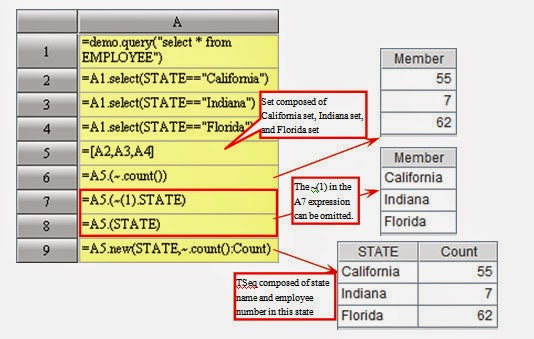7.Understanding Group

The group operation is commonly used in SQL. However, many people have no in-depth knowledge about it. The nature of a group operation is to split a set into several subsets according to a certain rule. In other words, the return value of a group operation shall be a set consisting of subsets. However, people often do not need to use such a set; instead, they may need to view a part of aggregate values of its subsets. Therefore, group operations are often followed by the summarization operations for subsets.

This is what the SQL does. The GROUP BY clause is always followed by a summarization operation. Because there are no explicit set data types in SQL, a set consisting of subsets cannot be returned directly. Therefore, summarization operations must follow group operations.

As time passes, one would think that the group operations are always accompanied with the follow-up summarization operations and forget that the group operations and the summarization operations are independently.

However, sometimes, we would still be interested in these grouped subsets but not the summarized values. Although a part of us might be interested in the summarized values, they would still need to hold these subsets for reuse, but not to discard them once a summarization is completed.

This requests us to understand the original meaning of the group operations. With a full realization of the thinking of sets, esProc can achieve this goal very well. In fact, the basic group functions in esProc can only be used for grouping which is independent to the summarization operation.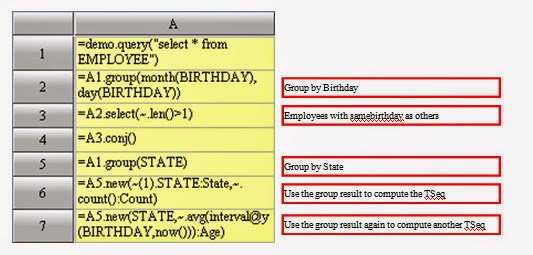The group result is a set consisting of several subsets, and the subset also can be grouped. Members in the group result are also sets; and they can be grouped.Both of the two operations will produce a multilayer set.Because these results are so deep in hierarchy, they are rarely used in practice. The above example is only to show you the thinking pattern of set and the nature of the operation.

8.Non-Equi Group

Besides the ordinary group function, esProc provides an align@a() function for processing alignment group and an enum() function for processing the enum group.

The group implemented by the group function is called an equi-group with the following features:

1) Any member in the original set must and can only be in one subset;
2) There is no empty subset.

The above two features are unavailable for the alignment group or the enumeration group.

Alignment group is an operation that calculates grouping expressions with members in a set and makes a match between resulting subsets and specified values. For the alignment group, the following steps are required:
1) Specify a group of values;
2) In the set to be grouped, move members whose property values are equal to the specified values to one subset;
3) Each resulting subset must be corresponding to a pre-defined condition.

It is possible that a member exists in none of subsets, an empty set exists, or any member exists in both subsets.

The following case will group employees by specified states sequence:Enum group: First, specify a group of conditions, take the members in the set to be grouped as arguments and compute these conditions, members satisfying these conditions will be grouped into a subset; each subset is corresponding to a pre-defined condition. A member may be in none of these subsets, or in two subsets at the same time; besides, an empty set may appear.

The following case will group employees by specified age groups:Although apparently it seams that these two functions differ greatly with the group, the three functions share the same nature regarding the group operation – to split a set into several sub-sets. The only difference is that these three functions split sets in different ways.